## A spring-loaded gun can fire a projectile to a height h if it is fired straight up. If the same gun is pointed at an angle of 45° from the v

Question

A spring-loaded gun can fire a projectile to a height h if it is fired straight up. If the same gun is pointed at an angle of 45° from the vertical, what maximum height can now be reached by the projectile? h4

in progress 0
2 months 2021-07-28T08:33:49+00:00 1 Answers 5 views 0

The maximum height can  be reached by the projectile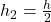Explanation:

Height = h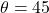Height in a projectile motion is given by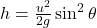When gun fired  straight up then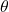= 90°

In that case Height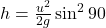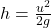—— (1)

When° then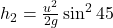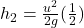From equation (1)This is the maximum height can  be reached by the projectile.